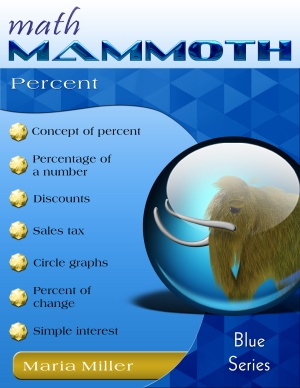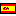GIVEAWAY! Win a Math Mammoth bundle or a grade level of your choice (13 winners!). Take part here. The giveaway runs through Sep 30, 2023.
^
You are here: HomeBlue Series → Percent

# Math Mammoth Percent80 pages

## Prices & ordering

Printed copy \$9.45 USDEste libro en español

Math Mammoth Percent teaches students the concept of percent, how to calculate the percentage of a number, to figure discounts, sales tax, and interest, to draw circle graphs, to differentiate between a percent of change and a percent of comparison, and to know how to calculate both. The text is suitable for grades 6 through 8 (middle school).

I have made several videos to match the lessons from this book. You can watch them here.

The concept of percent builds on the students' understanding of fractions and decimals. Specifically, students should be very familiar with the idea of finding a fractional part of a whole (such as finding 3/4 of \$240). Students who have used Math Mammoth have been practicing that concept since 4th grade. One reason why I have emphasized finding a fractional part of a whole so much in the earlier grades is specifically to lay a groundwork for the concept of percent. Assuming the student has mastered how to find a fractional part of a whole, and can easily convert fractions to decimals, then studying the concept of percent should not be difficult.

The first lesson, Percent, practices the concept of percent as a hundredth part, and how to write fractions and decimals as percentages. Next, we study how to find a percentage when the part and the whole are given (for example, if 15 out of 25 club members are girls, what percentage of them are girls?).

The following two lessons have to do with finding a certain percentage of a given number or quantity. First, we study how to do that using mental math techniques. For example, students find 10% of \$400 by dividing \$400 by 10. Next, students find a percentage of a quantity using decimal multiplication, including using a calculator. For example, students find 17% of 45 km by multiplying 0.17 × 45 km.

I prefer teaching students to calculate percentages of quantities using decimals, instead of using percent proportion or some other method (such as changing 17% into the fraction 17/100 for calculations). That is because using decimals is simpler: we simply change the percentage into a decimal, and multiply, instead of having to build a proportion or use fractions. Also, decimals will be so much easier to use later on, when solving word problems that require the usage of equations.

Next is a lesson about discounts, which is an important application in everyday life. Then, we go on to the lesson Practice with Percent, which contrasts the two types of problems students have already studied: questions that ask for a certain percentage of a number (the percentage is given), and questions that ask for the percentage. For example, the first type of question could be "What is 70% of \$380?", and the second type could be "What percentage is \$70 of \$380?"

Finding the Total When the Percent Is Known lets students find the total when the percentage and the partial amount are known. For example: "Three-hundred twenty students, which is 40% of all students, take PE. How many students are there in total?" We solve these with the help of bar models.

After a review lesson in the middle of the book, we study some of the basics again in the lessons Percentage and Solving Basic Percentage Problems. While the concepts are the same as in the lessons in the beginning of this book, this time we include more decimal digits and the coverage is faster, as these two lessons were originally written for 7th grade.

Percent Equations is meant for pre-algebra students and covers how to solve basic percent problems using an equation. It also explains the usage of a percent proportion.

The next major topic is the percentage of change, which is covered in a two-lesson sequence. The concept of percentage of change deals with percentage increases and decreases in quantities (especially prices). For example: "If an airline ticket that costs \$120 now goes up by 10%, then what will the new price be?" Students will also learn how to find an unknown percentage of change when the original and new quantities are known. For example, "If a shirt cost \$24 and is now discounted to \$18, then what percentage was the discount?"

Tying in with percentage of change, there is one lesson on Comparing Values Using Percentages. Students learn to solve comparisons involving percent (such as how many percent more (or less) one thing is than another) through applying concepts that they learned in finding the percentage of change and to differentiate clearly among the various types of comparison questions that can be asked.

Simple Interest is a lesson on the important topic of interest, using as a context both loans and savings accounts. Students learn to use the formula I = prt in a great variety of problems and situations.

The text concludes with a thorough review lesson of all of the concepts taught in the other lessons.

The PDF version of this book can be filled in on a computer, phone, or tablet, using the annotation tools found in many PDF apps. See more.

I have made several videos to match the lessons from this book. You can watch them here.

You might also be interested in:

I have never really been in a position to write one of those glowing testimonials that every product puts on their website, but I just had to tell you that I truly AM thrilled with Math Mammoth. Amanda just finished the Percent book in record time and actually seemed to enjoy it. In the course of two books, she has gone from super-frustrated, tightened-down, hating math of all kinds to deciding that it's not bad after all and just breezing through it. Practically miraculous, if you ask me. She thinks ratios are fun (Is this really my child??) so we are doing Ratios and Proportions next, and life is just a whole lot easier at this homeschool than it used to be.

Many thanks for a wonderful product that I can actually afford.

Blessings to you, Maria~

Ronda
See more testimonials

## Better Yet - Bundle Deals!

*BONUS*: Buy any bundle listed above, and get the fully upgraded Soft-Pak software for FREE (includes 4 math and 2 language arts programs, complete with on screen and printable options).

## How and where to order

You can buy Math Mammoth books at:

• Here at MathMammoth.com website — simply use the "Add to cart" buttons you see on the product pages.
• Rainbow Resource carries printed copies for the Light Blue series books, plus several CDs (Light Blue and Blue series).
• Homeschool Buyers Club offers download versions of the Light Blue series, plus the Blue series bundle.
• Homeschool Planet sells the digital versions of the main curriculum and lesson plans to go with them.
• Lulu sells printed copies for most of the Math Mammoth materials (various series).

By purchasing any of the books, permission IS granted for the teacher (or parent) to reproduce this material to be used with his/her students in a teaching situation; not for commercial resale. However, you are not permitted to share the material with another teacher.

In other words, you are permitted to make copies for the students/children you are teaching, but not for other teachers' usage.

Math Mammoth books are PDF files. I recommend you use Adobe Reader to view them, including if you use a Mac. You can try other PDF viewers, but they may at times either omit or mess up some of the images.
WAIT!

Receive my monthly collection of math tips & resources directly in your inbox — and get a FREE Math Mammoth book!You can unsubscribe at any time.

### Math Mammoth Tour

Confused about the different options? Take a virtual email tour around Math Mammoth! You'll receive:

An initial email to download your GIFT of over 400 free worksheets and sample pages from my books. Six other "TOURSTOP" emails that explain the important things and commonly asked questions concerning Math Mammoth curriculum. (Find out the differences between all these different-colored series!)

This way, you'll have time to digest the information over one or two weeks, plus an opportunity to ask me personally about the curriculum.
A monthly collection of math teaching tips & Math Mammoth updates (unsubscribe any time)### "Mini" Math Teaching Course

This is a little "virtual" 2-week course, where you will receive emails on important topics on teaching math, including:

- How to help a student who is behind
- Troubles with word problems
- Teaching multiplication tables
- Why fractions are so difficult
- The value of mistakes
- Should you use timed tests
- And more!

A GIFT of over 400 free worksheets and sample pages from my books right in the very beginning.A monthly collection of math teaching tips & Math Mammoth updates (unsubscribe any time)
Enter your email to receive math teaching tips, resources, Math Mammoth news & sales, humor, and more! I tend to send out these tips about once monthly, near the beginning of the month, but occasionally you may hear from me twice per month (and sometimes less often).• A GIFT of over 400 free worksheets and sample pages from my books.